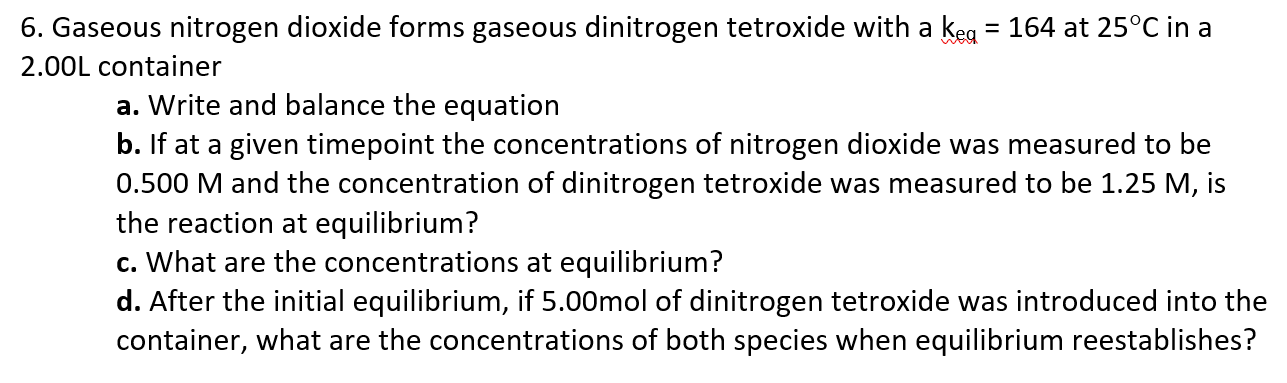# Gaseous nitrogen dioxide forms gaseous dinitrogen tetroxide with a keq = 164 at 25C in a 2.00L container a. Write and balance the equation b. If at a given timepoint the concentrations of nitrogen dioxide was measured to be 0.500 M and the concentration of dinitrogen tetroxide was measured to be 1.25 M, is the reaction at equilibrium? c. What are the concentrations at equilibrium? d. After the initial equilibrium, if 5.00mol of dinitrogen tetroxide was introduced into the container, what are